# Measurement Error Matrix at High Momentum

As shown in Section 3, in the high momentum limit, the r-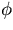and the r-z track fittings are decoupled so that the error matrix or its inverse corresponding to the coordinate measurement errors has a blockwise diagonal form: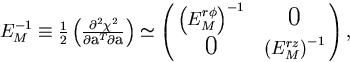(1)
where the component matrices are given by(2)
and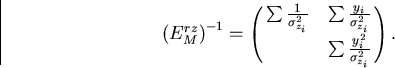(3)
Notice that, in this limit, the error matrix is determined completely by the y-locationsof the sampling points and the spatial resolutions thereat.

If (n+1) sampling points are equally spaced and have common resolutions,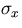and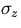, then the above equations become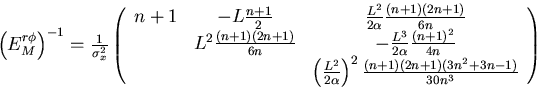(4)
and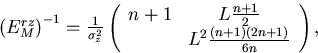(5)
where we have defined the lever arm length L by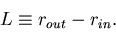(6)
Notice that in the above equations, we have shown only upper triangles of the matrices, since they are all symmetric. By matrix inversions, we thus obtain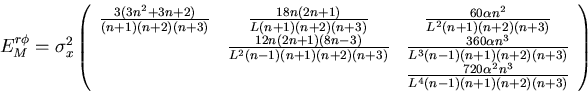(7)
and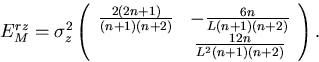(8)
The above error matrices are defined with the pivotal point (x0,y0,z0) chosen to be at the -th hit.

From the above formulae, we can estimate, for instance, the transverse momentum resolution or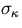as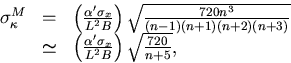(9)
where the last line is none other thatn the familiar text book expression for the momentum resolution valid in the large n limit.